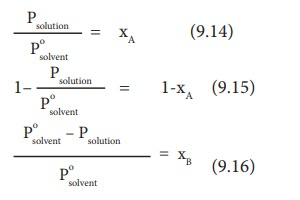Home | | Chemistry 11th std | Vapour pressure of binary solution of solids in liquids

# Vapour pressure of binary solution of solids in liquids

When a nonvolatile solute is dissolved in a pure solvent, the vapour pressure of the pure solvent will decrease.

Vapour pressure of binary solution of solids in liquids

When a nonvolatile solute is dissolved in a pure solvent, the vapour pressure of the pure solvent will decrease. In such solutions, the vapour pressure of the solution will depend only on the solvent molecules as the solute is nonvolatile.

For example, when sodium chloride is added to the water, the vapour pressure of the salt solution is lowered. The vapour pressure of the solution is determined by the number of molecules of the solvent present in the surface at any time and is proportional to the mole fraction of the solvent.Psolution ŌłØxA (9.11)

Where xA is the mole fraction of the solvent

Psolution    =   k xA  (9.12)

When xA  = 1,    K   = Posolvent

(Psolvento is the partial pressure of pure solvent)

Psolution    =   Posolvent xA  (9.13)Where xB is the fraction of the solute

(Ōł┤ xA + xB = 1, xB = 1 - xA)

The above expression gives the relative lowering of vapour pressure. Based on this expression, RaoultŌĆÖs Law can also be stated as ŌĆ£the relative lowering of vapour pressure of an ideal solution containing the nonvolatile solute is equal to the mole fraction of the solute at a given temperatureŌĆØ.## Comparison of RaoultŌĆÖs law and HenryŌĆÖs law

According to RaoultŌĆÖs law, for a solution containing a nonvolatile solute

psolute = p┬░solutexsolute (9.17)

According to HenryŌĆÖs law:

psolute = KHxsolute in solution (9.18)

The difference between the above two expressions is the proportionality constant p┬░A (Raoults Law) and KH .(Henry's Law). Henry's law is applicable to solution containing gaseous solute in liquid solvent, while the Raoults Law is applicable to nonvolatile solid solute in a liquid solvent. If the solute is non volatile then the Henry's law constant will become equal to the vapour of the pure solvent (p┬░) and thus, RaoultŌĆÖs law becomes a special Acase of HenryŌĆÖs law. For very dilute solutions the solvent obeys RaoultŌĆÖs law and the solute obeys HenryŌĆÖs law.

Study Material, Lecturing Notes, Assignment, Reference, Wiki description explanation, brief detail
11th Chemistry : UNIT 9 : Solutions : Vapour pressure of binary solution of solids in liquids |